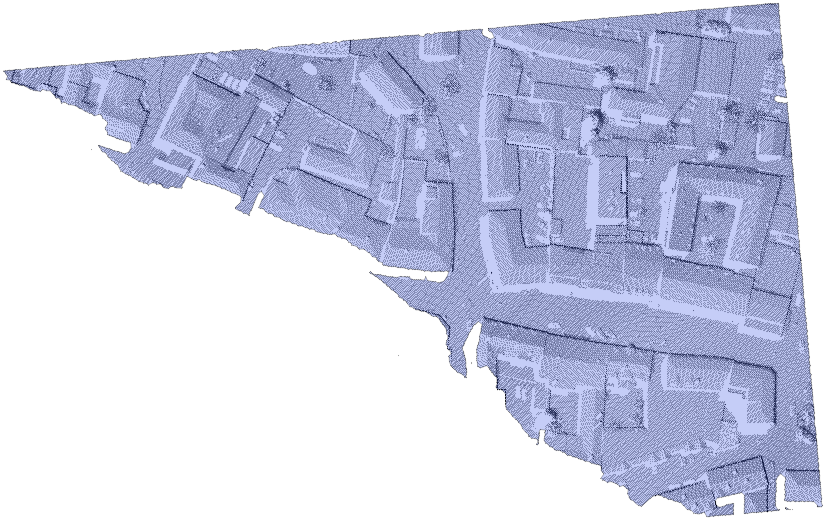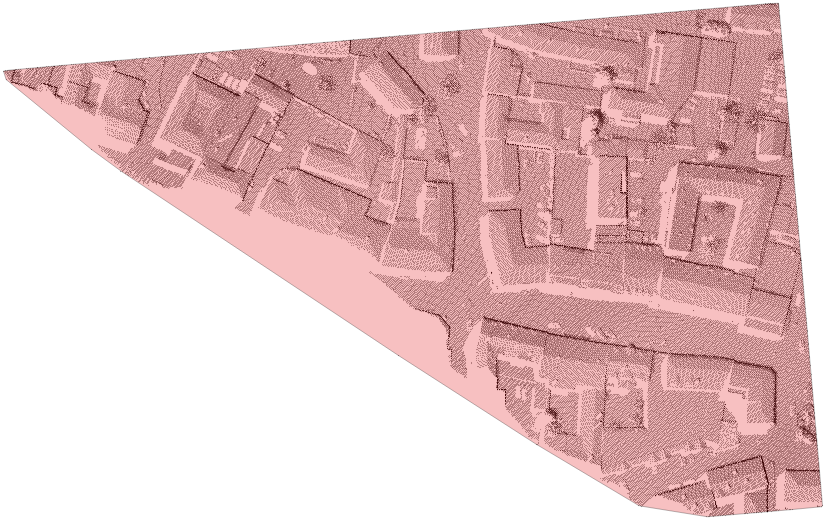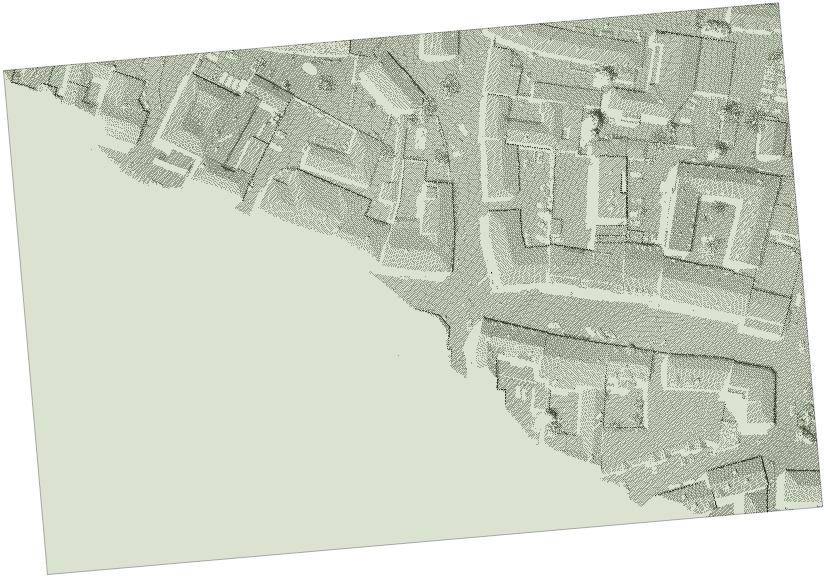Module Bounds

opals::IBounds

# Aim of module

Derives and stores the 2D boundary polygon of a region covered by the point data of an ODM or the valid pixels of a raster.

# General description

Even though the (vague) outline or shape of a point set may seem visually intuitive and clear, it may be defined and computed in various ways. The respective approaches may be classified into methods directly acting on the vector data and others that use rasterized versions of the data (thus depending on respective cell sizes). Module Bounds provides several methods that operate on vector data and derive a 2-dimensional outline of the point set provided by an ODM or a grid/raster. This outline is represented as 1 strictly simple (i.e. non-self-intersecting) polygon that may be convex or concave, depending on the method.

## Alpha-shapes

$$\alpha$$-shapes come close to what one would expect as a tight outline. $$\alpha$$-shapes are based on the triangulation of a point set. In regularized $$\alpha$$-shapes, as applied in Module Bounds, whole triangles together with their incident edges and vertices either belong to the shape or not, depending on the triangles' circumcircle radii, which must be smaller than the so-called $$\alpha$$-radius then. Therefore, the smaller the $$\alpha$$-radius, the fewer triangles form part of the $$\alpha$$-shape, and into the more connected components the $$\alpha$$-shape will be split. With increasing $$\alpha$$-radius, the $$\alpha$$-shape converges to the convex hull, constituting a single connected component only.

If the $$\alpha$$-radius is not specified, then Module Bounds uses the minimum $$\alpha$$-radius that makes all data points form part of the $$\alpha$$-shape and which yields a single connected component of $$\alpha$$-shape-triangles.

As the triangulation of large point sets is memory- and time-consuming, Module Bounds determines the coarse outline of data beforehand, and hence only triangulates the data within the outer regions. However, if point density varies largely within these regions, this approach may fail. In this case, a warning is emitted and the resulting $$\alpha$$-shape is the union of the computed $$\alpha$$-shape and the region omitted during triangulation. To avoid these failures, the parameter hollowingThresh may be set to a value larger than the number of data points: above this threshold, all points will be triangulated.

As a result, the outmost boundary of the $$\alpha$$-shape is used, being a possibly concave, but always strictly simple polygon.Outline derived with boundsType=alphaShape

## Convex hull

While the convex hull (generally) forms a coarser approximation to the data outline than $$\alpha$$-shapes do, this method is by far faster and features a much smaller memory footprint. As the name indicates, the resulting polygon is convex.Outline derived with boundsType=convexHull

## Minimum enclosing (oriented) rectangle

Based on the convex hull of the point set, this method derives the minimum rectangle that encloses all data points. This rectangle is oriented arbitrarily i.e. it is generally not axis-aligned.Outline derived with boundsType=minimumRectangle

# Parameter description

-inFileinput data manager or grid/raster file name
Type: opals::Path
Remarks: mandatory
Specify the path to the datamanager or grid/raster whose data bounds shall be determined.
-outFileoutput boundary file name
Type: opals::Path
Remarks: estimable
The boundary polygon will be saved to this file.
Estimation rule: [current directory] / [ODM name] + _bounds.[oformat_vector] .
-oFormatoutput boundary file format
Type: opals::String
Remarks: estimable
The boundary file will be exported using this format.
Estimation rule: default format for [oformat_vector] .
-boundsTypetype of boundary to compute
Type: opals::BoundaryType
Remarks: default=convexHull
Possible values:
convexHull ......... convex hull (strictly convex boundary polygon)
minimumRectangle ... minimum enclosing, oriented rectangle
alphaShape ......... alpha shape ( strictly simple boundary polygon with dished parts but without holes)

The type (level of detail) used for the derivation of the data boundary / outline. Alpha shapes are the most complex type representing the detailed data outline including concave as well as convex parts. The convex hull does not contain dished parts and the minimum (oriented) rectangle is the coarsest approximation of the data outline.
Type: double
Remarks: optional
In the determination of the alpha shape, triangles with a circumcircle radius larger than alphaRadius are removed consecutively, beginning at the convex hull.
If not given, use the minimum value that still leads to 1 connected component / polygon.
-hollowingThreshrelevant for alpha shapes: ODM point count below which all data are triangulated
Type: uint32
Remarks: default=500000
Above this point count, triangulate points near the outermost contour of data only.
If all points fit into memory, some problems may be avoided by specification of a high enough threshold.
-rasterTyperelevant for grid/raster input: force pixels to represent point or area
Type: opals::RasterType
Remarks: estimable
Possible values:
point ... pixel (center) represents a point, mainly used for DTM grids
area .... pixel represents area, mainly used for rater images like hill shading, etc.

If 'point' (grid), triangulate the pixel centers. If 'area' (raster), triangulate the pixel corners.
Estimation rule: use meta data, if available. Otherwise, use 'point'.

# Examples

The data used in the following example are located in the directory \$OPALS_ROOT/demo/. As the derivation of boundary lines relies on an ODM, the respective point cloud data has to be imported first. In this example, the dataset strip31.laz is used. To load the data into the ODM and rotate it counter-clockwise about the z-axis by 5°, change into the demo directory and type:

opalsImport -inf strip31.laz -filter "Affine[0.996 -0.087 0.087 0.996]"

In this example, only the mandatory parameter (inFile) is specified. Thus, the command is simply:

opalsBounds -inf strip31.odm

It produces the boundary file strip31_bounds.shp, using 'convexHull' as the default boundsType. The output is the boundary line as shown in the figure of subsection Convex hull.

Date
22.11.2011
opalsBounds is the executable file of Module Bounds
Definition: ModuleExecutables.hpp:18
opalsImport is the executable file of Module Import
Definition: ModuleExecutables.hpp:113
@ filter
string to be parsed in construction of DM::IFilter (various modules)